Courses

# Mosfet Electrical Engineering (EE) Notes | EduRev

## Electronic Devices

Created by: Cstoppers Instructors

## Electrical Engineering (EE) : Mosfet Electrical Engineering (EE) Notes | EduRev

The document Mosfet Electrical Engineering (EE) Notes | EduRev is a part of the Electrical Engineering (EE) Course Electronic Devices.
All you need of Electrical Engineering (EE) at this link: Electrical Engineering (EE)

MOSFET:-

We now turn our attention to the insulated gate FET or metal oxide semi-conductor FET which is of greater commercial importance than the junction FET.

Most MOSFETS however are triodes, with the substrate  internally connected to the source. The circuit symbols used by several manufacturers are indicated in the Fig below.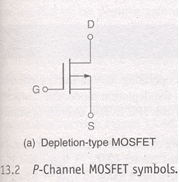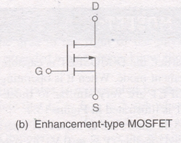(a) Depletion type MOSFET              (b) Enhancement type MOSFET

Both of them are P- channel

Here are two basic types of MOSFETS

(1) Depletion type           (2) Enhancement type MOSFET.

D-MOSFETS can be operated in both the depletion mode and the enhancement mode. E MOSFETS are restricted to operate in enhancement mode. The primary difference between them is their physical construction.

The construction difference between the two is shown in the fig given below.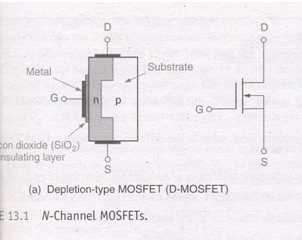As we can see the D-MOSFET have physical channel between the source and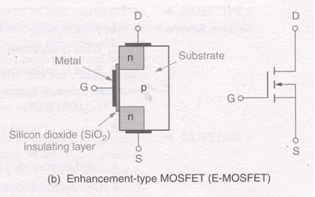The E-MOSFET on the other hand has no such channel physically. It depends on the gate voltage to form a channel between the source and the drain terminals.

Both MOSFETS have an insulating layer between the gate and the rest of the component. This insulating layer is made up of SiO2, a glass like insulating material. The gate material is made up of metal conductor .Thus going from gate to substrate, we can have metal oxide semi-conductor which is where the term MOSFET comes from.

Since the gate is insulated from the rest of the component, the MOSFET is sometimes referred to as an insulated gate FET or IGFET.

The foundation of the MOSFET is called the substrate. This material is represented in the schematic symbol by the center line that is connected to the source.

In the symbol for the MOSFET, the arrow is placed on the substrate. As with JFET an arrow pointing in represents an N-channel device, while an arrow pointing out represents P-channel device.

CONSTRUCTION OF AN N-CHANNEL MOSFET:-

The N- channel MOSFET consists of a lightly doped p-type substance into which two heavily doped n+ regions are diffused as shown in the Fig. These n+ sections , which will act as source and drain. A thin layer of insulation silicon dioxide (SiO2) is grown over the surface of the structure, and holes are cut into oxide layer, allowing contact with the source and drain. Then the gate metal area is overlaid on the oxide, covering the entire channel region. Metal contacts are made to drain and source and the contact to the metal over the channel area is the gate terminal.The metal area of the gate, in conjunction with the insulating dielectric oxide layer and the semiconductor channel, forms a parallel plate capacitor. The insulating layer of SiOis the reason why this device is called the insulated gate field effect transistor. This layer results in an extremely high input resistance (1010 to 1015 ohms) for MOSFET.

DEPLETION MOSFET

The basic structure of D–MOSFET is shown in the fig. An N-channel is diffused between source and drain with the device and appreciable drain current Idss flows for zero gate to source voltage, Vgs=0.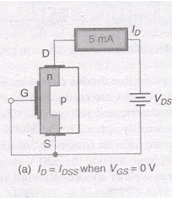Depletion mode operation:-

1. The above fig shows the D-MOSFET operating conditions with gate and source terminals shorted together (VGS=0V)
2. At this stage Id= Idss where VGS=0V, with this voltage Vds, an appreciable drain current Idss flows.
3. If the gate to source voltage is made negative i.e. Vgs is negative .Positive charges are induced in the channel through the SiO2 of the gate capacitor.
4. Since the current in a FET is due to majority carriers(electrons for an N-type material), the induced positive charges make the channel less conductive and the drain current drops as Vgs is made more negative.
5. The re-distribution of charge in the channel causes an effective depletion of majority carriers , which accounts for the designation depletion MOSFET.
6. That means biasing voltage Vgs depletes the channel of free carriers This effectively reduces the width of the channel , increasing its resistance.
7. Note that negative Vgs has the same effect on the MOSFET as it has on the JFET.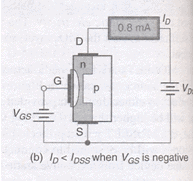1. As shown in the fig above, the depletion layer generated by Vgs (represented by the white space between the insulating material and the channel) cuts into the channel, reducing its width. As a result, Id<Idss. The actual value of Id depends on the value of Idss,Vgs(off) and Vgs.

Enhancement mode operation of the D-MOSFET:-

1. This operating mode is a result of applying a positive gate to source voltage Vgs to the device.
2. When Vgs is positive the channel is effectively widened.  This reduces the resistance of the channel allowing Id to exceed the value of Idss.
3. When Vgs is given positive the majority carriers in the p-type are holes. The holes in the p-type substrate are repelled by the +ve gate voltage.
4. At the same time, the conduction band electrons (minority carriers) in the p-type material are attracted towards the channel by the +ve gate voltage.
5. With the build up of electrons near the channel , the area to the right of the physical channel effectively becomes an N-type material.
6. The extended n-type channel now allows more current, Id> Idss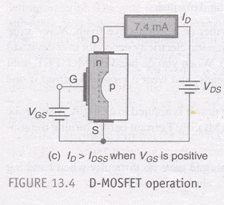Characteristics of Depletion MOSFET:-

The fig. shows the drain characteristics for the N-channel depletion type MOSFET

1. The curves are plotted for both positive and negative values of Vgs
2. When Vgs=0 and negative the MOSFET operates in depletion mode and when Vgs is positive ,the MOSFET operates in the enhancement mode.
3. The difference between JFET and D-MOSFET is that JFET does not operate for positive values of Vgs.
4. When Vds=0, there is no conduction between source to drain. If Vgs<0 and Vds>0 then Id increases linearly.
5. But as Vgs>0 induces positive charges holes in the channel, and controls the channel width. Thus the conduction between source to drain is maintained as constant, i.e. Id is constant.
6. If Vgs>0, the gate induces more electrons in channel side, it is added with the free electrons generated by source. Again the potential applied to gate determines the channel width and maintains constant current flow through it as shown in figure.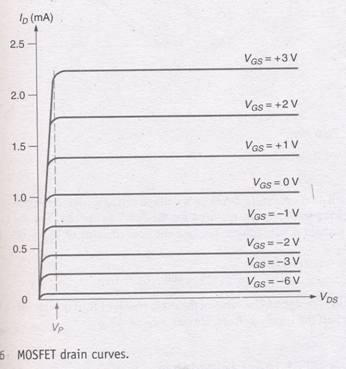TRANSFER CHARACTERISTICS:-

The combination of 3 operating states i.e. Vgs=0V, Vgs<0V, Vgs>0V is represented by the D-MOSFET transconductance curve shown in Fig.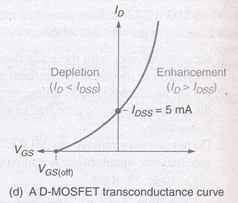1. Here in this curve it may be noted that the region AB of the characteristics similar to that of JFET.
2. This curve extends for the positive values of Vgs.
3. Note that Id=Idss for Vgs=0V when Vgs is negative,Id< Idss when Vgs= Vgs(off) ,Id is reduced to approximately omA. Where Vgs is positive Id>Idss. So obviously Idss is not the maximum possible value of Id for a MOSFET.
4. The curves are similar to JFET so that the D-MOSFET have the same transconductance equation.

E-MOSFETS

The E-MOSFET is capable of operating only in the enhancement mode. The gate potential must be positive w.r.t to source.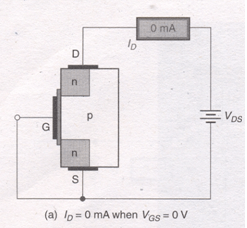1. when the value of Vgs=0V, there is no channel connecting the source and drain materials.
2. As a result , there can be no significant amount of drain current.
3. When Vgs=0, the Vdd supply tries to force free electrons from source to drain but the presence of p-region does not permit the electrons to pass through it. Thus there is no drain current at Vgs=0.
4. If Vgs is  positive, it induces a negative charge in the p type substrate just adjacent to the SiO2 layer.
5. As the holes are repelled by the positive gate voltage, the minority carrier electrons attracted toward this voltage. This forms an effective N-type bridge between source and drain providing a path for drain current.
6. This +ve gate voltage forms a channel between the source and drain.
7. This produces a thin layer of N-type channel in the P-type substrate. This layer of free electrons is called N-type inversion layer.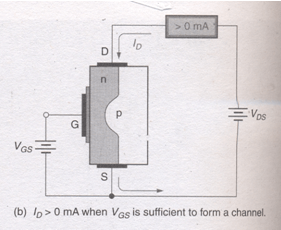8.  The minimum Vgs which produces this inversion layer is called threshold voltage and is designated by Vgs(th). This is the point at which the device turns on is called the threshold voltage Vgs(th).
9.  When the voltage Vgs is <Vgs (th), no current flows from drain to source.
10. However when the voltage Vgs > Vgs(th) the inversion layer connects the drain to source and we get significant values of current.

CHARACTERISTICS OF E MOSFET:-

1. DRAIN CHARACTERISTICS

The volt ampere drain characteristics of an N-channel enhancement mode MOSFET are given in the figure.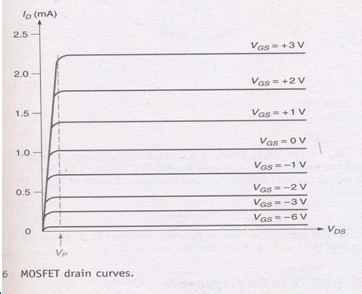1. TRANSFER CHARACTERISTICS:-
2. The current Idss at Vgs≤ 0 is very small being of the order of a few nano amps.
3. As Vgs is made +ve , the current Id increases slowly at first, and then much more rapidly with an increase in Vgs.
4. The standard transconductance formula will not work for the E-MOSFET.
5. To determine the value of Id at a given value of Vgs we must use the following relation-

Id =K[Vgs-Vgs(Th)]2

Where K is constant for the MOSFET . found as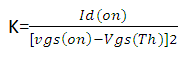From the data specification sheets, the 2N7000 has the following ratings.

Id(on)= 75mA(minimum).

And     Vgs(th)=0.8(minimum)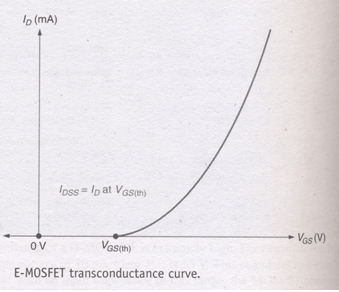6.8 APPLICATION OF MOSFET

One of the primary contributions to electronics made by  MOSFETs  can be found in the area of digital (computer electronics). The signals in digital circuits are made up of rapidly switching dc levels. This signal is known as a rectangular wave ,made up of two dc levels (or logic levels). These logic levels are 0V and +5V.

A group of circuits with similar circuitry and operating characteristics is referred to as a logic family. All the circuits in a given logic family respond to the same logic levels, have similar speed and power-handling capabilities  , and can be directly connected together. One such logic family is complementary MOS (or CMOS)  logic. This logic family is made up entirely of  MOSFETs.

Offer running on EduRev: Apply code STAYHOME200 to get INR 200 off on our premium plan EduRev Infinity!

,

,

,

,

,

,

,

,

,

,

,

,

,

,

,

,

,

,

,

,

,

;